### Common Notation

Table 70.29 displays notation for some of the more common parameters across analyses. The Associated Syntax column shows examples of relevant analysis statement options, where applicable.

Table 70.29 Common Notation

Symbol

Description

Associated SyntaxSignificance level

ALPHA=
Total sample size

NTOTAL=, NPAIRS=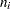Sample size inth group

NPERGROUP=, GROUPNS=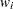Allocation weight forth group (standardized to sum to 1)

GROUPWEIGHTS=(Arithmetic) mean

MEAN=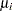(Arithmetic) mean inth group

GROUPMEANS=, PAIREDMEANS=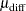(Arithmetic) mean difference,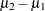or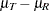MEANDIFF=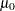Null mean or mean difference (arithmetic)

NULL=, NULLDIFF=
Geometric mean

MEAN=
Geometric mean inth group

GROUPMEANS=, PAIREDMEANS=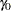Null mean or mean ratio (geometric)

NULL=, NULLRATIO=
Standard deviation (or common standard deviation per group)

STDDEV=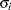Standard deviation inth group

GROUPSTDDEVS=, PAIREDSTDDEVS=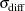Standard deviation of differences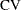Coefficient of variation, defined as the ratio of the standard deviation to the (arithmetic) mean

CV=, PAIREDCVS=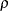Correlation

CORR=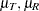Treatment and reference (arithmetic) means for equivalence test

GROUPMEANS=, PAIREDMEANS=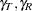Treatment and reference geometric means for equivalence test

GROUPMEANS=, PAIREDMEANS=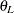Lower equivalence bound

LOWER=
Upper equivalence bound

UPPER=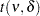distribution with d.f.and noncentrality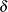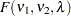distribution with numerator d.f.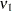, denominator d.f.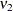, and noncentrality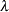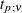th percentile ofdistribution with d.f.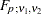th percentile ofdistribution with numerator d.f.and denominator d.f.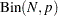Binomial distribution with sample sizeand proportionA "lower one-sided" test is associated with SIDES=L (or SIDES=1 with the effect smaller than the null value), and an "upper one-sided" test is associated with SIDES=U (or SIDES=1 with the effect larger than the null value).

Owen (1965) defines a function, known as Owen’s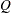, that is convenient for representing terms in power formulas for confidence intervals and equivalence tests: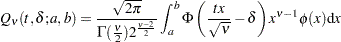where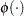and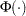are the density and cumulative distribution function of the standard normal distribution, respectively.